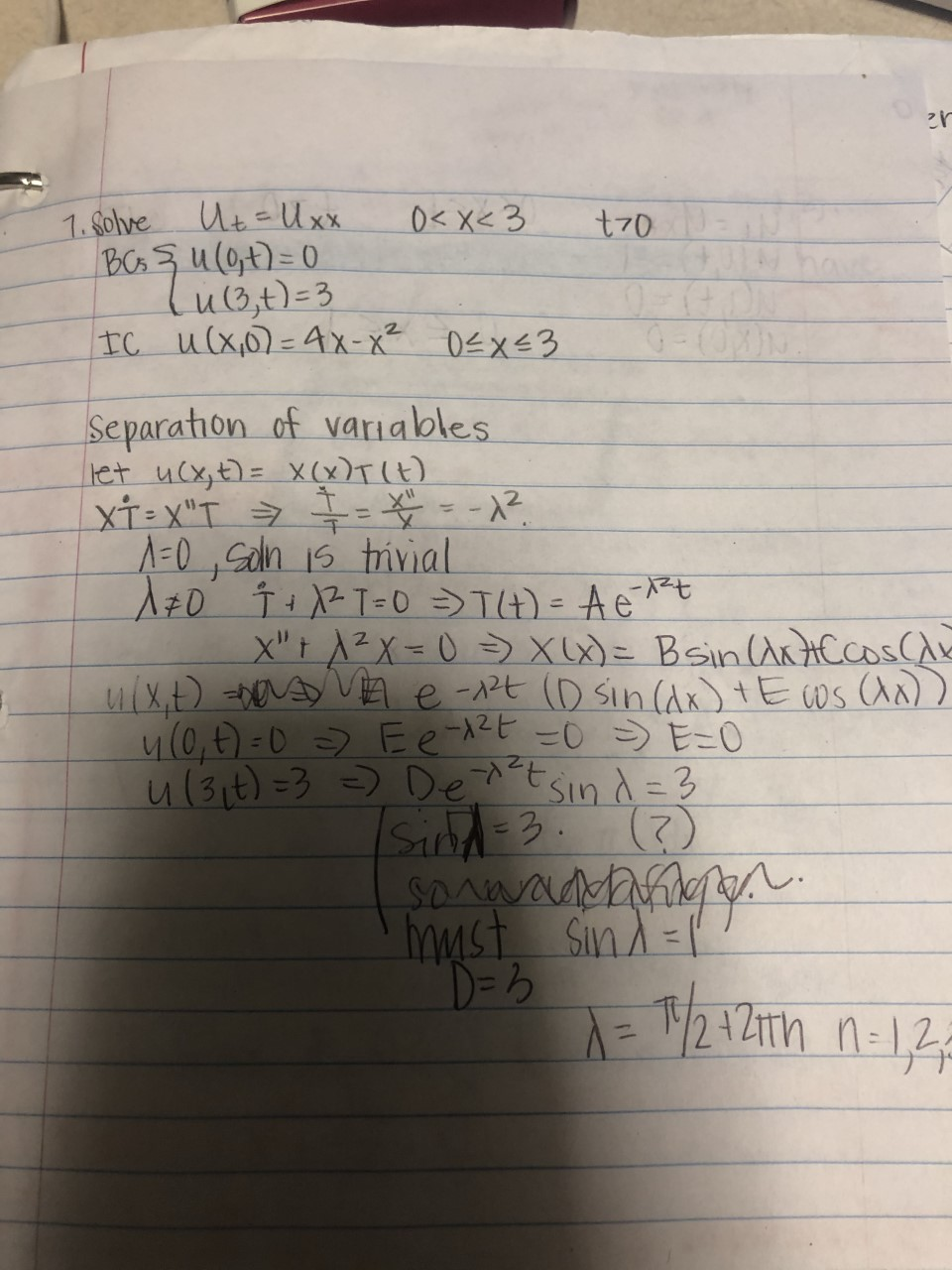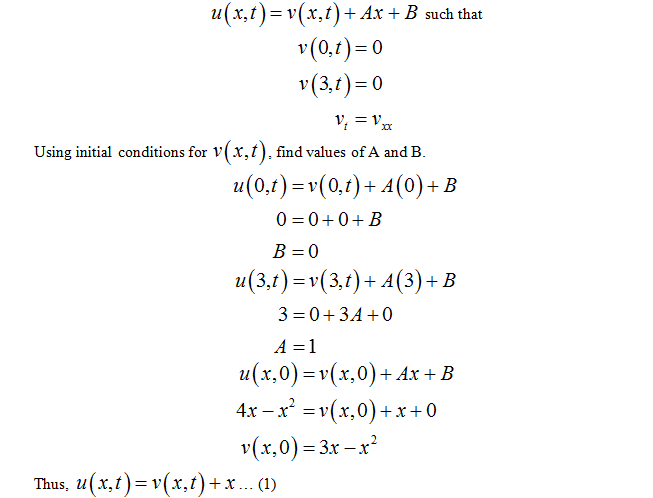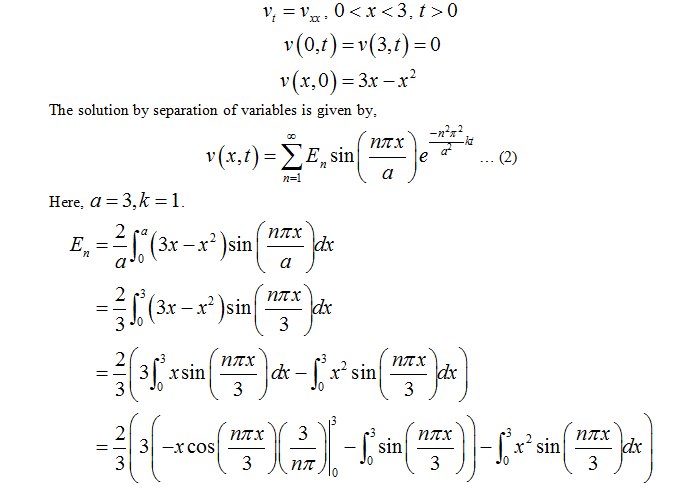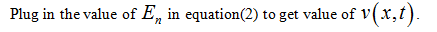# 1. Solve Ut-Ux.BCs şu(0t)=D0lu1Bt)=3IC u(x0)=4x-x² 0T4) = AetX"t A²X=0 => xu)= Bsin ArtACCoS(Aulx.t) basy VA e-2t (D sin(Ax) tE ws (An))4(0,E) =0 > Ee 12E -0>E-0Sin d= 3Sand=3. ()SDAWAADMSind=l%3Du(3it) =3 =) De^tmystD=3%3D

Question
1 views

Partial Differential Equation

by separation of variables.  I have begun but am stuck

Plz & thank you!!help_outlineImage Transcriptionclose1. Solve Ut-Ux. BCs şu(0t)=D0 lu1Bt)=3 IC u(x0)=4x-x² 0T4) = Aet X"t A²X=0 => xu)= Bsin ArtACCoS(A ulx.t) basy VA e-2t (D sin(Ax) tE ws (An)) 4(0,E) =0 > Ee 12E -0>E-0 Sin d= 3 Sand=3. () SDAWAADM Sind=l %3D u(3it) =3 =) De^t myst D=3 %3D fullscreen
check_circle

star
star
star
star
star
1 Rating
Step 1 Given equation is a heat equation with non-homogeneous boundary conditions.

In case of non-homogeneous boundary conditions, we apply the transformation,Step 2 Now, the new heat equation with homogeneous boundary conditions is:Step 3 Solve for En by applying integral by parts to second integrand as well....

### Want to see the full answer?

See Solution

#### Want to see this answer and more?

Solutions are written by subject experts who are available 24/7. Questions are typically answered within 1 hour.*

See Solution
*Response times may vary by subject and question.
Tagged in